# Binary Search Trees Notes | Study GATE Computer Science Engineering(CSE) 2023 Mock Test Series - GATE

## GATE: Binary Search Trees Notes | Study GATE Computer Science Engineering(CSE) 2023 Mock Test Series - GATE

The document Binary Search Trees Notes | Study GATE Computer Science Engineering(CSE) 2023 Mock Test Series - GATE is a part of the GATE Course GATE Computer Science Engineering(CSE) 2023 Mock Test Series.
All you need of GATE at this link: GATE

Binary Search Tree | (Search and Insertion)

The following is definition of Binary Search Tree(BST) according to Wikipedia

Binary Search Tree, is a node-based binary tree data structure which has the following properties:

• The left subtree of a node contains only nodes with keys less than the node’s key.
• The right subtree of a node contains only nodes with keys greater than the node’s key.
• The left and right subtree each must also be a binary search tree.
There must be no duplicate nodes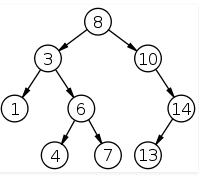The above properties of Binary Search Tree provide an ordering among keys so that the operations like search, minimum and maximum can be done fast. If there is no ordering, then we may have to compare every key to search a given key.

Searching a key

To search a given key in Bianry Search Tree, we first compare it with root, if the key is present at root, we return root. If key is greater than root’s key, we recur for right subtree of root node. Otherwise we recur for left subtree.

// C function to search a given key in a given BST
struct node* search(struct node* root, int key)
{
// Base Cases: root is null or key is present at root
if (root == NULL || root->key == key)
return root;

// Key is greater than root's key
if (root->key < key)
return search(root->right, key);

// Key is smaller than root's key
return search(root->left, key);
}

Illustration: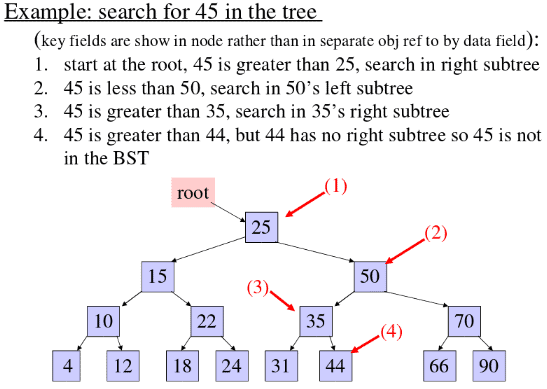Insertion of a key

A new key is always inserted at leaf. We start searching a key from root till we hit a leaf node. Once a leaf node is found, the new node is added as a child of the leaf node.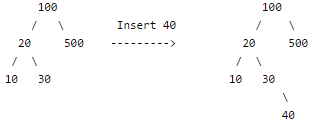// C program to demonstrate insert operation in binary search tree
#include<stdio.h>
#include<stdlib.h>

struct node
{
int key;
struct node *left, *right;
};

// A utility function to create a new BST node
struct node *newNode(int item)
{
struct node *temp =  (struct node *)malloc(sizeof(struct node));
temp->key = item;
temp->left = temp->right = NULL;
return temp;
}

// A utility function to do inorder traversal of BST
void inorder(struct node *root)
{
if (root != NULL)
{
inorder(root->left);
printf("%d ", root->key);
inorder(root->right);
}
}

/* A utility function to insert a new node with given key in BST */
struct node* insert(struct node* node, int key)
{
/* If the tree is empty, return a new node */
if (node == NULL) return newNode(key);

/* Otherwise, recur down the tree */
if (key < node->key)
node->left  = insert(node->left, key);
else if (key > node->key)
node->right = insert(node->right, key);

/* return the (unchanged) node pointer */
return node;
}

// Driver Program to test above functions
int main()
{
/* Let us create following BST
50
/
30      70
/      /
20   40  60   80 */
struct node *root = NULL;
root = insert(root, 50);
insert(root, 30);
insert(root, 20);
insert(root, 40);
insert(root, 70);
insert(root, 60);
insert(root, 80);

// print inoder traversal of the BST
inorder(root);

return 0;
}

Output:
20
30
40
50
60
70
80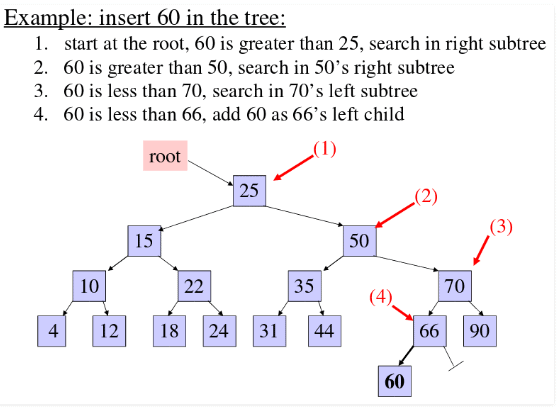## Binary Search Tree | (Delete)

We have discussed BST search and insert operations. In this post, delete operation is discussed. When we delete a node, there possibilities arise.

1) Node to be deleted is leaf: Simply remove from the tree.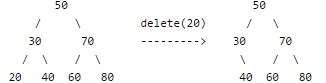2) Node to be deleted has only one child: Copy the child to the node and delete the child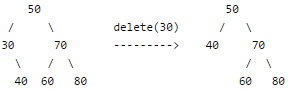3) Node to be deleted has two children: Find inorder successor of the node. Copy contents of the inorder successor to the node and delete the inorder successor. Note that inorder predecessor can also be used.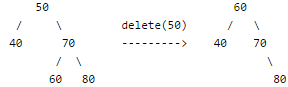The important thing to note is, inorder successor is needed only when right child is not empty. In this particular case, inorder successor can be obtained by finding the minimum value in right child of the node.

// C program to demonstrate delete operation in binary search tree
#include<stdio.h>
#include<stdlib.h>

struct node
{
int key;
struct node *left, *right;
};

// A utility function to create a new BST node
struct node *newNode(int item)
{
struct node *temp =  (struct node *)malloc(sizeof(struct node));
temp->key = item;
temp->left = temp->right = NULL;
return temp;
}

// A utility function to do inorder traversal of BST
void inorder(struct node *root)
{
if (root != NULL)
{
inorder(root->left);
printf("%d ", root->key);
inorder(root->right);
}
}

/* A utility function to insert a new node with given key in BST */
struct node* insert(struct node* node, int key)
{
/* If the tree is empty, return a new node */
if (node == NULL) return newNode(key);

/* Otherwise, recur down the tree */
if (key < node->key)
node->left  = insert(node->left, key);
else
node->right = insert(node->right, key);

/* return the (unchanged) node pointer */
return node;
}

/* Given a non-empty binary search tree, return the node with minimum
key value found in that tree. Note that the entire tree does not
need to be searched. */
struct node * minValueNode(struct node* node)
{
struct node* current = node;

/* loop down to find the leftmost leaf */
while (current->left != NULL)
current = current->left;

return current;
}

/* Given a binary search tree and a key, this function deletes the key
and returns the new root */
struct node* deleteNode(struct node* root, int key)
{
// base case
if (root == NULL) return root;

// If the key to be deleted is smaller than the root's key,
// then it lies in left subtree
if (key < root->key)
root->left = deleteNode(root->left, key);

// If the key to be deleted is greater than the root's key,
// then it lies in right subtree
else if (key > root->key)
root->right = deleteNode(root->right, key);

// if key is same as root's key, then This is the node
// to be deleted
else
{
// node with only one child or no child
if (root->left == NULL)
{
struct node *temp = root->right;
free(root);
return temp;
}
else if (root->right == NULL)
{
struct node *temp = root->left;
free(root);
return temp;
}

// node with two children: Get the inorder successor (smallest
// in the right subtree)
struct node* temp = minValueNode(root->right);

// Copy the inorder successor's content to this node
root->key = temp->key;

// Delete the inorder successor
root->right = deleteNode(root->right, temp->key);
}
return root;
}

// Driver Program to test above functions
int main()
{
/* Let us create following BST
50
/
30      70
/      /
20   40  60   80 */
struct node *root = NULL;
root = insert(root, 50);
root = insert(root, 30);
root = insert(root, 20);
root = insert(root, 40);
root = insert(root, 70);
root = insert(root, 60);
root = insert(root, 80);

printf("Inorder traversal of the given tree ");
inorder(root);

printf(" Delete 20 ");
root = deleteNode(root, 20);
printf("Inorder traversal of the modified tree ");
inorder(root);

printf(" Delete 30 ");
root = deleteNode(root, 30);
printf("Inorder traversal of the modified tree ");
inorder(root);

printf(" Delete 50 ");
root = deleteNode(root, 50);
printf("Inorder traversal of the modified tree ");
inorder(root);

return 0;
}

Output:

Inorder traversal of the given tree
20 30 40 50 60 70 80
Delete 20
Inorder traversal of the modified tree
30 40 50 60 70 80
Delete 30
Inorder traversal of the modified tree
40 50 60 70 80
Delete 50
Inorder traversal of the modified tree
40 60 70 80

Illustration: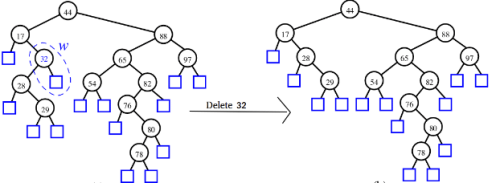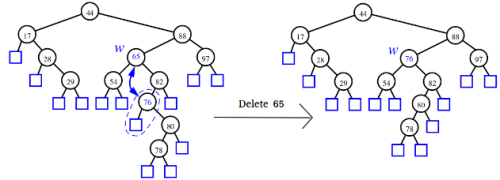## A program to check if a binary tree is BST or not

A binary search tree (BST) is a node based binary tree data structure which has the following properties.
• The left subtree of a node contains only nodes with keys less than the node’s key.
• The right subtree of a node contains only nodes with keys greater than the node’s key.
• Both the left and right subtrees must also be binary search trees.

From the above properties it naturally follows that:
• Each node (item in the tree) has a distinct key.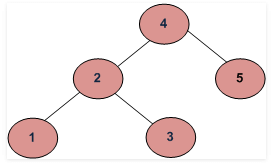METHOD 1 (Simple but Wrong)
Following is a simple program. For each node, check if left node of it is smaller than the node and right node of it is greater than the node.

int isBST(struct node* node)

if (node == NULL)
return 1;

/* false if left is > than node */
if (node->left != NULL && node->left->data > node->data)
return 0;

/* false if right is < than node */
if (node->right != NULL && node->right->data < node->data)
return 0;

/* false if, recursively, the left or right is not a BST */
if (!isBST(node->left) || !isBST(node->right))
return 0;

/* passing all that, it's a BST */
return 1;
}

This approach is wrong as this will return true for below binary tree (and below tree is not a BST because 4 is in left subtree of 3)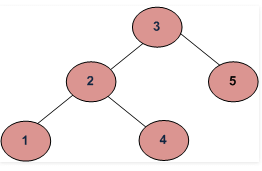METHOD 2 (Correct but not efficient)
For each node, check if max value in left subtree is smaller than the node and min value in right subtree greater than the node.

/* Returns true if a binary tree is a binary search tree */
int isBST(struct node* node)

if (node == NULL)
return(true);

/* false if the max of the left is > than us */
if (node->left!=NULL && maxValue(node->left) > node->data)
return(false);

/* false if the min of the right is <= than us */
if (node->right!=NULL && minValue(node->right) < node->data)
return(false);

/* false if, recursively, the left or right is not a BST */
if (!isBST(node->left) || !isBST(node->right))
return(false);

/* passing all that, it's a BST */
return(true);
}

It is assumed that you have helper functions minValue() and maxValue() that return the min or max int value from a non-empty tree

METHOD 3 (Correct and Efficient)
Method 2 above runs slowly since it traverses over some parts of the tree many times. A better solution looks at each node only once. The trick is to write a utility helper function isBSTUtil(struct node* node, int min, int max) that traverses down the tree keeping track of the narrowing min and max allowed values as it goes, looking at each node only once. The initial values for min and max should be INT_MIN and INT_MAX — they narrow from there.

/* Returns true if the given tree is a binary search tree
(efficient version). */
int isBST(struct node* node)

return(isBSTUtil(node, INT_MIN, INT_MAX));

/* Returns true if the given tree is a BST and its
values are >= min and <= max. */
int isBSTUtil(struct node* node, int min, int max)

Implementation:

#include <stdio.h>
#include <stdlib.h>
#include <limits.h>

/* A binary tree node has data, pointer to left child
and a pointer to right child */
struct node
{
int data;
struct node* left;
struct node* right;
};

int isBSTUtil(struct node* node, int min, int max);

/* Returns true if the given tree is a binary search tree
(efficient version). */
int isBST(struct node* node)

return(isBSTUtil(node, INT_MIN, INT_MAX));

/* Returns true if the given tree is a BST and its
values are >= min and <= max. */
int isBSTUtil(struct node* node, int min, int max)

/* an empty tree is BST */
if (node==NULL)
return 1;

/* false if this node violates the min/max constraint */
if (node->data < min || node->data > max)
return 0;

/* otherwise check the subtrees recursively,
tightening the min or max constraint */
return
isBSTUtil(node->left, min, node->data-1) &&  // Allow only distinct values
isBSTUtil(node->right, node->data+1, max);  // Allow only distinct values

/* Helper function that allocates a new node with the
given data and NULL left and right pointers. */
struct node* newNode(int data)
{
struct node* node = (struct node*)
malloc(sizeof(struct node));
node->data = data;
node->left = NULL;
node->right = NULL;

return(node);
}

/* Driver program to test above functions*/
int main()
{
struct node *root = newNode(4);
root->left        = newNode(2);
root->right       = newNode(5);
root->left->left  = newNode(1);
root->left->right = newNode(3);

if(isBST(root))
printf("Is BST");
else
printf("Not a BST");

getchar();
return 0;
}

Time Complexity: O(n)
Auxiliary Space : O(1) if Function Call Stack size is not considered, otherwise O(n)

METHOD 4(Using In-Order Traversal)
1) Do In-Order Traversal of the given tree and store the result in a temp array.
3) Check if the temp array is sorted in ascending order, if it is, then the tree is BST.

Time Complexity: O(n)

We can avoid the use of Auxiliary Array. While doing In-Order traversal, we can keep track of previously visited node. If the value of the currently visited node is less than the previous value, then tree is not BST.

bool isBST(struct node* root)
{
static struct node *prev = NULL;

// traverse the tree in inorder fashion and keep track of prev node
if (root)
{
if (!isBST(root->left))
return false;

// Allows only distinct valued nodes
if (prev != NULL && root->data <= prev->data)
return false;

prev = root;

return isBST(root->right);
}

return true;
}

## Find the node with minimum value in a Binary Search Tree

This is quite simple. Just traverse the node from root to left recursively until left is NULL. The node whose left is NULL is the node with minimum value.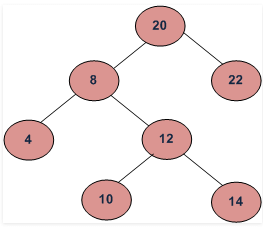For the above tree, we start with 20, then we move left 8, we keep on moving to left until we see NULL. Since left of 4 is NULL, 4 is the node with minimum value.

#include <stdio.h>
#include<stdlib.h>

/* A binary tree node has data, pointer to left child
and a pointer to right child */
struct node
{
int data;
struct node* left;
struct node* right;
};

/* Helper function that allocates a new node
with the given data and NULL left and right
pointers. */
struct node* newNode(int data)
{
struct node* node = (struct node*)
malloc(sizeof(struct node));
node->data  = data;
node->left  = NULL;
node->right = NULL;

return(node);
}

/* Give a binary search tree and a number,
inserts a new node with the given number in
the correct place in the tree. Returns the new
root pointer which the caller should then use
(the standard trick to avoid using reference
parameters). */
struct node* insert(struct node* node, int data)
{
/* 1. If the tree is empty, return a new,
single node */
if (node == NULL)
return(newNode(data));
else
{
/* 2. Otherwise, recur down the tree */
if (data <= node->data)
node->left  = insert(node->left, data);
else
node->right = insert(node->right, data);

/* return the (unchanged) node pointer */
return node;
}
}

/* Given a non-empty binary search tree,
return the minimum data value found in that
tree. Note that the entire tree does not need
to be searched. */
int minValue(struct node* node) {
struct node* current = node;

/* loop down to find the leftmost leaf */
while (current->left != NULL) {
current = current->left;
}
return(current->data);
}

/* Driver program to test sameTree function*/
int main()
{
struct node* root = NULL;
root = insert(root, 4);
insert(root, 2);
insert(root, 1);
insert(root, 3);
insert(root, 6);
insert(root, 5);

printf(" Minimum value in BST is %d", minValue(root));
getchar();
return 0;
}

## Advantages of BST over Hash Table

Hash Table supports following operations in Θ(1) time.
1) Search
2) Insert
3) Delete

The time complexity of above operations in a self-balancing Binary Search Tree (BST) (like  Red-Black Tree, AVL Tree Splay Tree, etc) is O(Logn).

So Hash Table seems to beating BST in all common operations. When should we prefer BST over Hash Tables, what are advantages. Following are some important points in favor of BSTs.

1. We can get all keys in sorted order by just doing Inorder Traversal of BST. This is not a natural operation in Hash Tables and requires extra efforts.
2. Doing Order Statistics, finding closest lower and qreater elementns, doing range querise are easy to do with BSTs. Like sorting, these operations are not a natural operation with Hash Tables.
3. BSTs are easy to implement compared to hashing, we can easily implement our own customized BST. To implement Hashing, we generally rely on libraries provided by programming languages.
4. With BSTs, all operations are guaranteed to work in O(Logn) time. But with Hashing, Θ(1) is average time and some particular operations may be costly, especially when table resizing happens.

Time Complexity: O(n) Worst case happens for left skewed trees.

Similarly we can get the maximum value by recursively traversing the right node of a binary search tree.

The document Binary Search Trees Notes | Study GATE Computer Science Engineering(CSE) 2023 Mock Test Series - GATE is a part of the GATE Course GATE Computer Science Engineering(CSE) 2023 Mock Test Series.
All you need of GATE at this link: GATEUse Code STAYHOME200 and get INR 200 additional OFF

## GATE Computer Science Engineering(CSE) 2023 Mock Test Series

136 docs|165 tests

Track your progress, build streaks, highlight & save important lessons and more!

,

,

,

,

,

,

,

,

,

,

,

,

,

,

,

,

,

,

,

,

,

;